# Periodic Functions

A function f (x) is called periodic if it is defined for all real ‚Äûx‚Äü some positive number ‚Äûp‚Äü such that f (x + p ) = f (x) for all x.

PERIODIC FUNCTIONS

A function f (x) is called periodic if it is defined for all real ‚Äûx‚Äü some positive number ‚Äûp‚Äü such that

f (x + p ) = f (x) for all x.

This         number   ‚Äûp‚Äü   is   called   a   period   of   f(x

If a periodic function f (x) has a smallest period p (>0), this is often called the fundamental period of f(x). For example, the functions cosx and sinx have fundamental period 2p.

DIRICHLET CONDITIONS

Any function f(x), defined in the interval c ¬£¬£c + 2p, can be developed as a Fourier series of the form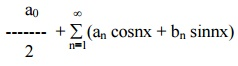provided the following conditions are satisfied.

f (x) is periodic, single‚Äìvalued and finite in [ c, c + 2 p].

f (x) has a finite number of discontinuities in [ c, c + 2p].

f (x) has at the most a finite number of maxima and minima in [ c,c+ 2p].

These conditions are known as Dirichlet conditions. When these conditions are satisfied, the Fourier series converges to f(x) at every point of continuity. At a point of discontinuity x = c, the sum of the series is given by

f(x) = (1/2) [ f (c-0) + f (c+0)] ,

where f (c-0) is the limit on the left and f (c+0) is the limit on the right.

EULER‚ÄôS  FORMULAE

The Fourier series for the function f(x) in the interval c < x < c + 2pis given byThese values of a0, an, bn are   known   as   Euler‚Äüs 0 , formula en,b The coefficients a0, an, bn  are  are also termed as Fourier coefficients.

Example 1

Expand f(x) = x as Fourier Series (Fs)  in the interval [ -œÄ,   œÄ]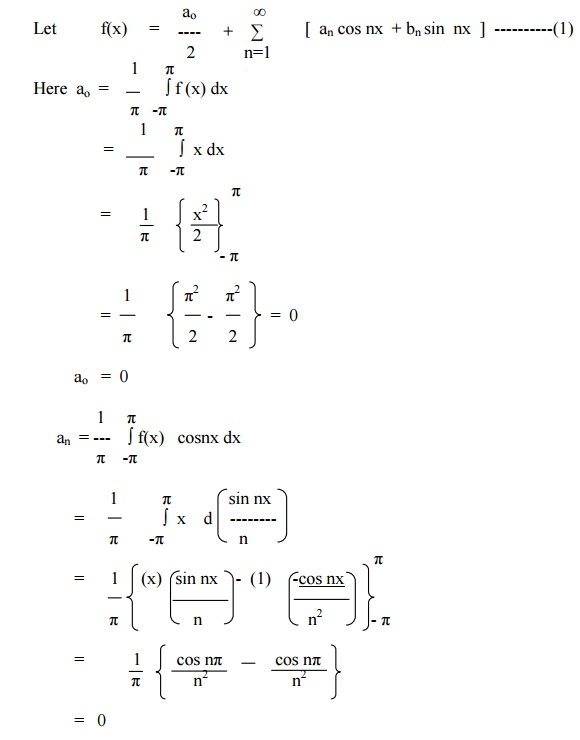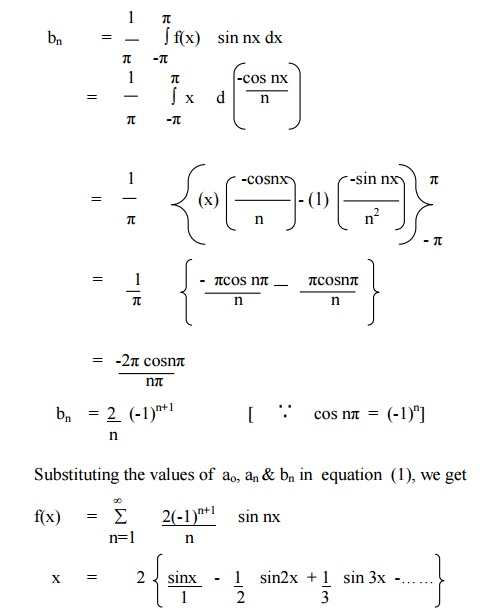Example 2

Expand   f(x) = xin the interval ( -p¬£¬£p) and hence deduce thatExample 3

Obtain the Fourier Series of periodicity   2œÄin [for-œÄ, œÄ]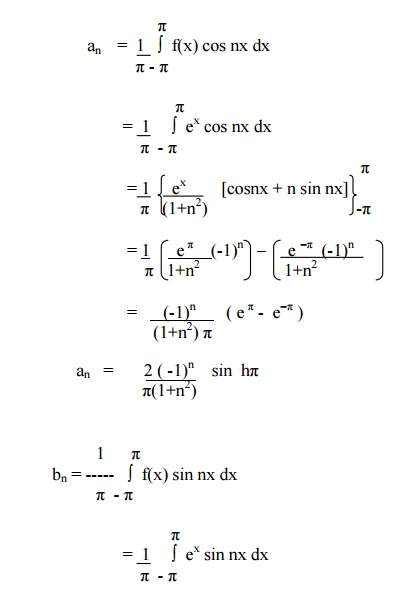Example 4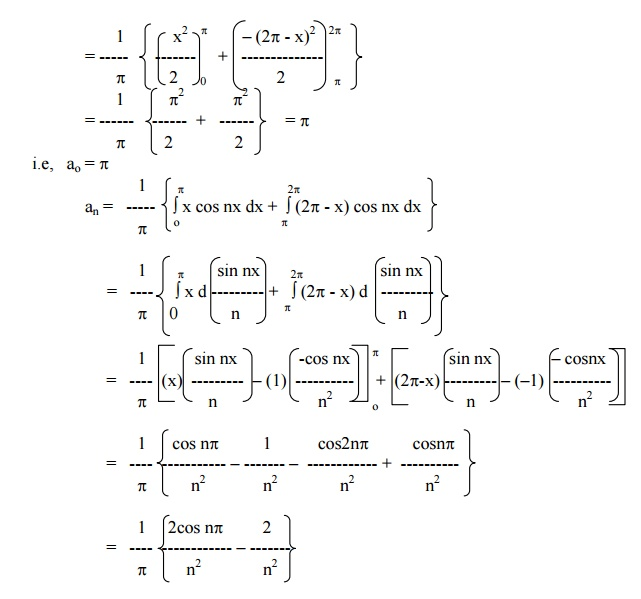Example 5

Find the Fourier series for f (x) = (x + x2) in (-p< x < p) of percodicity 2and hence deduce thatHere x = -pand x = pare the end points of the range. \ The value of FS at x = pis the average of the values of f(x) at x = pand x = -p.Exercises:

Determine the Fourier expressions of the following functions in the given interval

1.f(x) = (p- x)2, 0 < x < 2p

2.f(x) = 0 in -p< x < 0

pin 0 < x < p

3.f(x) = x ‚Äìx2 in [-p,p

4.f(x) = x(2p-x) in (0,2p

5.f(x) = sinh ax in [-pp

6.f(x) = cosh ax in [-pp

7.f(x) = 1 in 0 < x < p

= 2 in p< x < 2p

8.f(x) = -p/4 when -p< x < 0

=  p/4 when 0 < x < p

9.f(x) = cosax, in -p< x < p,   wherea‚Äüis‚Äû not   an   integer

10.Obtain a fourier series to represent e-ax from x = -pto x = p. Hence derive the series for p/ sinhp

Study Material, Lecturing Notes, Assignment, Reference, Wiki description explanation, brief detail
Mathematics (maths) : Fourier Series : Periodic Functions |